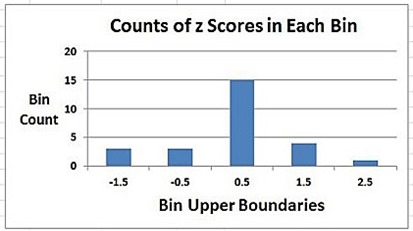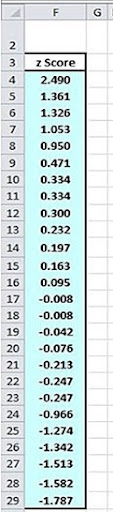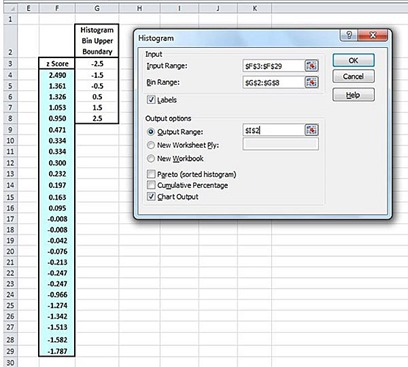## Tuesday, May 27, 2014

### Histogram With the Histogram Data Analysis Tool in Excel

This is one of the following two articles on creating Histograms in Excel

Creating a Histogram With the Histogram Data Analysis Tool in Excel

Creating an Automatically Updating Histogram in 7 Steps in Excel With Formulas and a Bar Chart

# Overview of Histograms in Excel

A histogram provides the counts of the number of items in groups after the items have been divided into groups of similar items. In statistics the items are divided into groups based upon the value of a continuous variable that can be measured for each item. The value of that variable for each item determines which group the item will fall into.

Each group only accepts items that have variable values in a specific and unique range. The groups are sometimes referred to as bins. A histogram provides the counts of the number of items that have fallen into each bin. Each bin only accepts items within a specific range of variable values.

Histograms are often presented in the form of bar charts. Each bar is associated with a unique bin. The length of each bar represents the number items in the bin associated with that bar.

Adjacent bars on the histogram bar chart are associated with bins that have adjacent ranges for accepting items. The histogram bar chart therefore shows how all of the items are distributed or divided up based on the variable value measured for each item. An example of a histogram is as follows:(Click On the Image To See an Enlarged Version)

This histogram provides count of the number of items that have z Scores in each of the five categories or bins. The first bin on the right accepted items that have a z Score between 2.5 and 1.5. Only one item has been observed to have a z Score in that range. The second bin to the left shows that four items have been observed to have z Scores between 0.5 and 1.5.

The bell-shaped curve of the histogram indicates that the z Score might be normally distributed. A histogram provides a quick visual estimation of a variable’s distribution.

The preceding histogram was taken from the following data. This histogram shows how the 26 z Scores in the sample group are distributed. The bell-shaped histogram indicates that these z Score might be normally distributed. The bell-shaped histogram results from the groups or bins that accept z Scores in the middle ranges having have significantly higher counts than bins that accept z Scores in the outer ranges.

An important component of a histogram is establishing the upper and lower boundaries of ranges that each bin will accept. The following images shows data sample of 26 z Scores, the upper and lower ranges of each of the five bins, the count of z Scores falling into each bin, and the bar chart histogram that provides a graphically display of the bin counts.(Click On the Image To See an Enlarged Version)

## Steps To Take Before Creating a Histogram

Data that will be evaluated with a histogram are often not provided in pre-determined bins. The bins, or more specifically, the upper and lower boundaries of each of the bins, have not been established. Sorting and standardizing the data greatly facilitates the development of the bin specifications.

Sorting the data makes the data’s range and any significant outliers apparent. Outliers judged to be extreme and therefore non-representative of the data can be removed. Each significant outlier should be evaluated on a case-by-case basis. Outliers that have been removed and the justifications for removal should be noted.

Standardizing a data value converts that value to its z Score. A z Score is equal to the number of sample standard deviations that the value is from the sample mean. Standardizing the data allow bin boundaries to be based upon increments of sample standard deviation which is fairly intuitive and uncomplicated.

An example of sorting and standardizing a sample of raw data is performed as follows:

Unsorted Raw Data Sample### a) Sorting the Data

The raw data can be sorted using the sorting tool available in Excel. This is effective but an even better way to sort the data is use the formula shown in the following diagram. Using this formula has the advantage that the data will be automatically resorted if any of the data are changed. The sorting tool would need to be reapplied each time any of the data have been changed. The formula can be typed into the top cell and then quickly copied down to the bottom as follows:(Click On the Image To See an Enlarged Version)

### b) Standardizing the Data

Standardizing the data simply involves subtracting the mean from the data value and then dividing by the standard deviation. This calculation converts each data value to its z Score. For population data, the z Score is the number of population standard deviations that the data value is from the population mean. For sample data, the z Score is the number of sample standard deviations that a data value is from the sample mean.

The z Scores in this example are calculated from sample data as follows:(Click On the Image To See an Enlarged Version)

The raw data sample has been sorted and standardized to produce the following sorted sample of 26 z Scores.(Click On the Image To See an Enlarged Version)

### c) Creating the Bins

Bin creation involves specifying the upper and lower boundaries of each bin into which the values will fall. Creating optimal bins ranges depends on the skill of the user. The bins ranges should be large enough that at least several observations are likely to be in each bin but not so large that the shape of the data’s distribution shape cannot be ascertained because too many observations have fallen into too few bins. Ultimately there needs to be a sufficiently large number of observations collected to enable the creation of a reasonably clear distribution shape with a histogram.

The z Scores of the data range from -1.787 to 2.490. The bins should cover that entire range because there are no significant outliers among the 26 total z Scores. One possible configuration of bins ranges is the following set of five adjacent bins each having the width equal to one z Score as follows:(Click On the Image To See an Enlarged Version)

## The Two Methods To Create a Histogram in Excel

There are two ways to create a histogram in Excel. Histograms will be created in this section using both of these methods.

The first method uses Excel’s built-in Histogram tool that is part of the Data Analysis ToolPak. The Data Analysis ToolPak ships with most versions of Excel but need to be manual activated by the user before it is available for use. Like all of the Data Analysis tool, the Histogram tool must be manually rerun to update the histogram if any of the input data has changed.

The second method combines Excel formulas and a bar chart to create a histogram. This is the preferred method because the histogram will be automatically updated if any of the input data has changed. Excel formulas and chart automatically adjust their output when input data is changed. This method of creating a histogram in Excel will be demonstrated in detail in the blog article just before this one.

It is sometimes more efficient to use formulas in place of the Data Analysis tools because the formula automatically update the output when input data is changed. Substituting formulas for Data Analysis tools is only a good solution for simple tools such the Histogram tool but not complicated tools such as Two-Way ANOVA With Replication tool or the Multiple Regression tool.

## Creating a Histogram With the Excel Histogram Data Analysis Tool

The Excel Histogram tool requires that the bin ranges be specified by providing only the upper boundary of each bin. The Histogram tool dialogue box can be accessed in Excel under the Data tab by selecting Data Analysis / Histogram. The Histogram dialogue box then appears. The data range, bin upper boundaries, upper left corner of the output, and chart Output checkmark are input into this dialogue box as follows:(Click On the Image To See an Enlarged Version)

The specified histogram output which includes the frequency chart and the histogram bar chart as shown as follows:(Click On the Image To See an Enlarged Version)

Note that the frequency chart requires the creation of a bin above and below the five bins that are the target for this histogram analysis. This is not intuitive and usually requires some experimentation to properly create the five bin ranges that are the target of this analysis. Another shortcoming of the Histogram tool in the Data Analysis ToolPak is that the histogram must be re-run whenever input data has changed.

These two shortcomings of the Histogram tool can be overcome by creating the histogram with Excel formulas and a bar chart. Creating a histogram in Excel with a bar chart and formulas will be demonstrated in the blog article next to this one.

Excel Master Series Blog Directory

Statistical Topics and Articles In Each Topic

• Histograms in Excel
• Bar Chart in Excel
• Combinations & Permutations in Excel
• Normal Distribution in Excel
• t-Distribution in Excel
• Binomial Distribution in Excel
• z-Tests in Excel
• t-Tests in Excel
• Hypothesis Tests of Proportion in Excel
• Chi-Square Independence Tests in Excel
• Chi-Square Goodness-Of-Fit Tests in Excel
• F Tests in Excel
• Correlation in Excel
• Pearson Correlation in Excel
• Spearman Correlation in Excel
• Confidence Intervals in Excel
• Simple Linear Regression in Excel
• Multiple Linear Regression in Excel
• Logistic Regression in Excel
• Single-Factor ANOVA in Excel
• Two-Factor ANOVA With Replication in Excel
• Two-Factor ANOVA Without Replication in Excel
• Randomized Block Design ANOVA in Excel
• Repeated-Measures ANOVA in Excel
• ANCOVA in Excel
• Normality Testing in Excel
• Nonparametric Testing in Excel
• Post Hoc Testing in Excel
• Creating Interactive Graphs of Statistical Distributions in Excel
• Solving Problems With Other Distributions in Excel
• Optimization With Excel Solver
• Chi-Square Population Variance Test in Excel
• Analyzing Data With Pivot Tables
• SEO Functions in Excel
• Time Series Analysis in Excel
• VLOOKUP

1.Very useful thank you.
And maybe this could help everybody to learn Ms Excel further

Excel Data Analysis For Dummies-For Dummies (2014).pdf

2.Every assignment expert need to know creating Histograms in Excel so he/she can solve Excel assignments, so it is an very useful information for me. Thanks.

3.Get the service for your law assignment help UK task because of it is very famous for your college level assignment.

4.After the objects have been classified into groups of comparable items, a histogram provides counts of the number of items in each category. In statistics, items purchase coursework online
are classified as either large or little.

5.We have the quality centric, and tailored sociology dissertation help, our sociology dissertation editors will ensure your dissertation and give their best.

6.I ordered my thesis at essay professors https://essaysprofessors.com We wrote a good work. There were no comments from my teacher.

7.I love your blog. I always find something interesting here. Recently a friend of mine showed me https://elitewritings.com/buy-a-letter-of-recommendation.html letter of recommendation writing service. This service is great. I can get a letter of recommendation. I can save my time.

8.Histograms in Excel are basically graphical representation of data acquired by the program, such as percentage of normals, the number of different colors, average values and other data. This type of graphical data is useful for situations where you need Saving Gain Coupons to summarize data from a number of different observations

9.A maroon leather moto jacket is a Luxury, durable outfit with a zippered front and fitted or elastic waist and cuffs. Available at your doorstep, order now from topcelebsjackets.com.

10.Nobody wants to start the day discovering that their car’s air conditioning is not working, the bad air-conditioning not only effect your driving comfort it also affect your car performance as well? Depending on the make and model of your car, a broken belt in the air conditioner can cause more problems for your vehicle. Do not wait until it’s too late! If it’s as simple asCheck engine light service Abu Dhabi recharging the refrigerant or installing a new compressor, then ALSA Automotive Repair will help keep you cool and your care cool.

11.We treat our clients like family so we ensure when you rely on us, like family, we will not let you down! We will help you at Buy Assignment Uk - Assignmentdone.co.uk We are pleased to state that till date there is not a single customer who was left disappointed with our service.